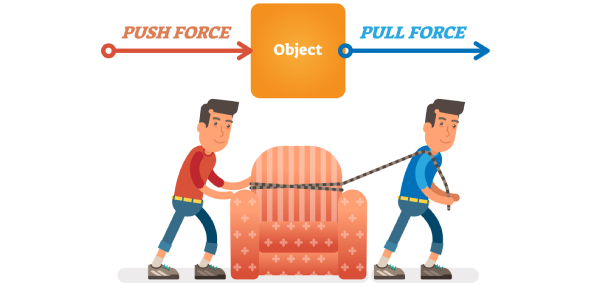# Force And Motion Quiz For 3rd Grade Students

Approved & Edited by ProProfs Editorial Team
The editorial team at ProProfs Quizzes consists of a select group of subject experts, trivia writers, and quiz masters who have authored over 10,000 quizzes taken by more than 100 million users. This team includes our in-house seasoned quiz moderators and subject matter experts. Our editorial experts, spread across the world, are rigorously trained using our comprehensive guidelines to ensure that you receive the highest quality quizzes.
| By Tgrayson
T
Tgrayson
Community Contributor
Quizzes Created: 1 | Total Attempts: 4,525
Questions: 9 | Attempts: 4,558Settings• 1.

### The place where someone or something is placed

• A.

Position

• B.

System

• C.

Physical property

• D.

Over there

A. Position
Explanation
The correct answer is "position" because it refers to the place where someone or something is located. It is a term commonly used to describe the location or placement of an object or individual in relation to their surroundings.

Rate this question:

• 2.

### The force that pulls us and everything around us down

• A.

Magnetic

• B.

Motion

• C.

Repel

• D.

Gravity

D. Gravity
Explanation
Gravity is the force that pulls us and everything around us down. It is a fundamental force of nature that exists between any two objects with mass. Unlike magnetic forces, which can attract or repel depending on the orientation of the objects, gravity always attracts objects towards each other. It is responsible for keeping us grounded on the Earth and governs the motion of celestial bodies in the universe.

Rate this question:

• 3.

### To draw or pull toward an object

• A.

Force

• B.

Gravity

• C.

Attract

• D.

Push

C. Attract
Explanation
The word "attract" means to draw or pull toward an object. It is the action of exerting a force that brings objects closer together.

Rate this question:

• 4.

### A change in position

• A.

Push

• B.

Pull

• C.

Speed

• D.

Motion

D. Motion
Explanation
Motion refers to a change in position of an object over time. It can be described as the movement or displacement of an object from one place to another. In this context, the other options (push, pull, and speed) are not as comprehensive as motion in describing a change in position. While push and pull can cause motion, they do not encompass all types of motion. Speed, on the other hand, is a measure of how fast an object is moving but does not capture the concept of a change in position itself. Therefore, motion is the most fitting answer.

Rate this question:

• 5.

### The measure of the pull of gravity on an object

• A.

Work

• B.

Weight

• C.

Repel

• D.

Speed

B. Weight
Explanation
Weight is the measure of the pull of gravity on an object. It is the force with which an object is attracted towards the center of the Earth or any other celestial body. Weight is determined by the mass of the object and the acceleration due to gravity. It is commonly measured in units of force, such as pounds or newtons.

Rate this question:

• 6.

### The measure of force that it takes to move an object a certain distance

• A.

Work

• B.

Force

• C.

Speed

• D.

Motion

A. Work
Explanation
Work is the correct answer because it refers to the measure of force required to move an object a certain distance. Work is calculated by multiplying force and displacement, and it is the transfer of energy that occurs when an object is moved against a force. Therefore, work is the appropriate term to describe the measure of force required to move an object a certain distance.

Rate this question:

• 7.

### To force backward

• A.

Gravity

• B.

Repel

• C.

Push

• D.

Pull

B. Repel
Explanation
The term "repel" means to push away or to cause something to move in the opposite direction. In the context of the given question, "to force backward," it can be inferred that the force being referred to is causing something to move away or be pushed in the opposite direction. Therefore, "repel" is the correct answer as it aligns with the meaning of the term and the given context.

Rate this question:

• 8.

### To push or pull

• A.

Force

• B.

Motion

• C.

Work

• D.

Position

A. Force
Explanation
Force is the correct answer because it is the term that encompasses both pushing and pulling. Force is a physical quantity that can cause an object to move, change its motion, or stay in a particular position. It is a fundamental concept in physics and is measured in units such as Newtons. Whether an object is being pushed or pulled, a force is being applied to it to cause a change in its state of motion or position.

Rate this question:

• 9.

### A set of parts or elements forming a whole and functioning as a unit

• A.

System

• B.

Work

• C.

Force

• D.

Gravity

A. System
Explanation
A system is a set of parts or elements that work together as a whole and function as a unit. It implies that the individual components are interconnected and interdependent, with each part contributing to the overall functioning and behavior of the system. This term is commonly used in various fields, such as science, engineering, and technology, to describe complex arrangements or organizations that exhibit specific properties or behaviors.

Rate this question:

Related TopicsBack to top